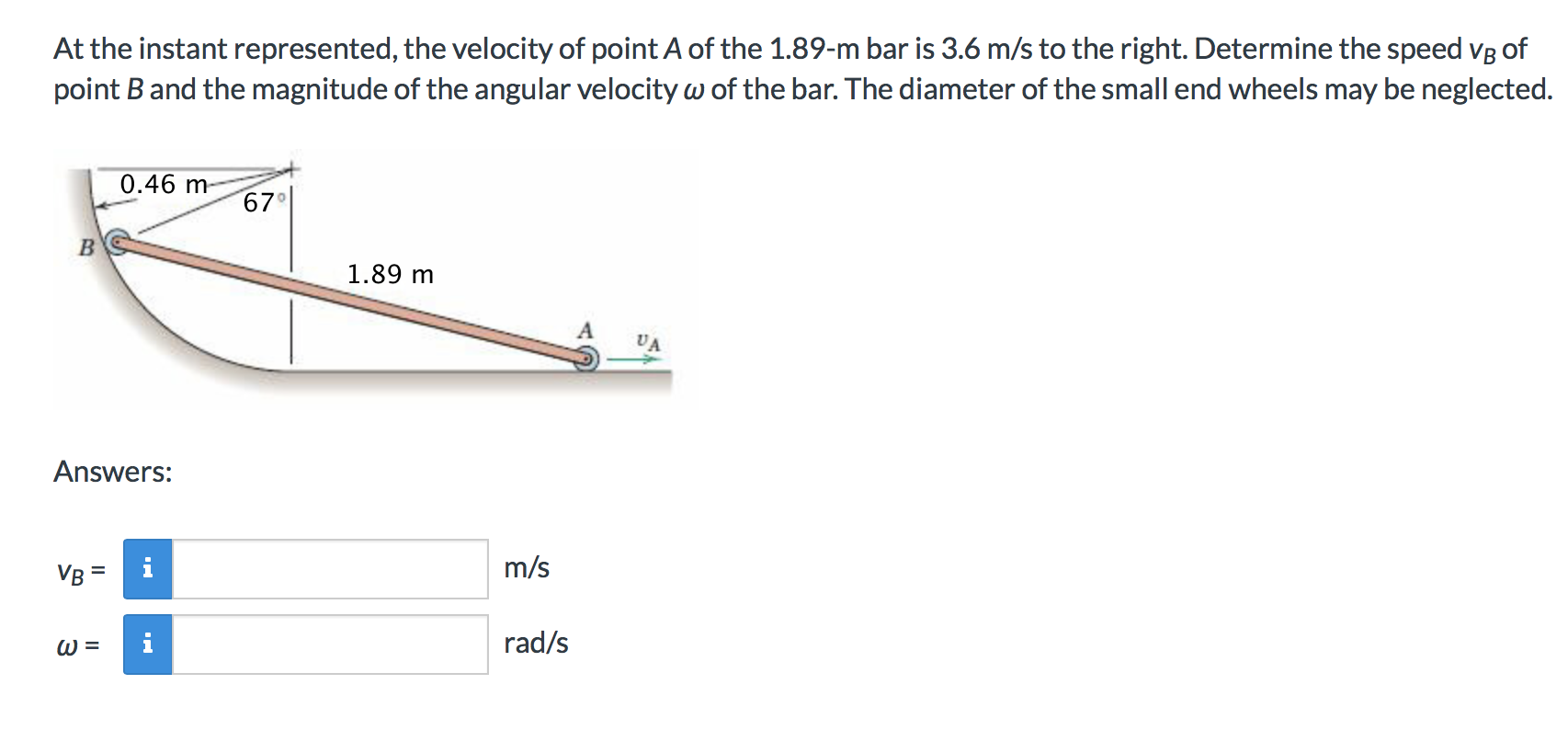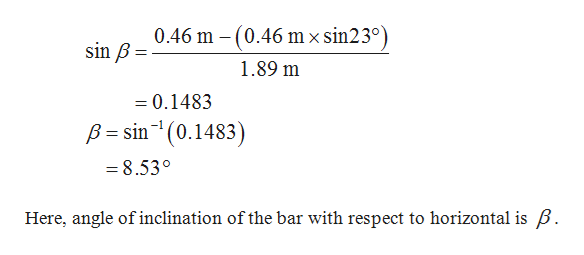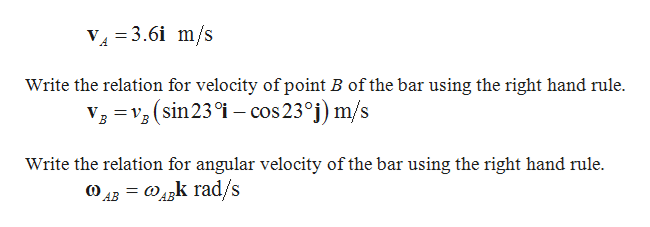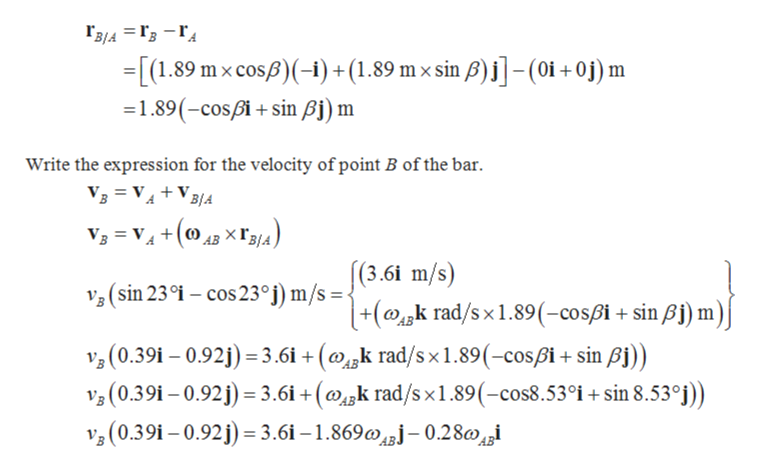# At the instant represented, the velocity of point A of the 1.89-m bar is 3.6 m/s to the right. Determine the speed VB ofpoint B and the magnitude of the angular velocity w of the bar. The diameter of the small end wheels may be neglected.0.46 m-67B1.89 mAnswers:m/sVBrad/s

Questionhelp_outlineImage TranscriptioncloseAt the instant represented, the velocity of point A of the 1.89-m bar is 3.6 m/s to the right. Determine the speed VB of point B and the magnitude of the angular velocity w of the bar. The diameter of the small end wheels may be neglected. 0.46 m- 67 B 1.89 m Answers: m/s VB rad/s fullscreen
check_circleExpert Solution
Step 1

Calculate the angle of inclination of the bar with respect to horizontal.help_outlineImage Transcriptionclose0.46 m (0.46 m x sin230) sin B= 1.89 m =0.1483 Bsin(0.1483) =8.53° Here, angle of inclination of the bar with respect to horizontal is 6 fullscreen
Step 2

Write the relation for velocity of point A of the bar using the right hand rule.help_outlineImage TranscriptioncloseV 3.6i m/s Write the relation for velocity of point B of the bar using the right hand rule v3 V (sin 23i - cos23°j) m/s Write the relation for angular velocity of the bar using the right hand rule. k rad/s fullscreen
Step 3

Calculate the position vector of point B with resp...help_outlineImage TranscriptioncloseГз4 3 Гз — Гд [(1.89 mx cosp(-) + (1.89 mx sin )-(oi+ oj) m =1.89(-cospisin Bj) m Write the expression for the velocity of point B of the bar Vз 3D Vд+V34 3= V+()XIg) [(3.6i m/s) 3(sin 23°i - cos 23°j) m/s=\ +(k rad/s x 1.89 (-cospi + sin Bj) m)j 3(0.391 - 0.92j 3.61 + (krad/sx1.89(-cospi+ sin Aj)) 3 (0.39i -0.92) 3.61+ (k rad/sx1.89(-cos8.53°j+sin 8.53°j)) v3 (0.39i-0.92 3.61-1.869j-0.28i fullscreen

### Want to see the full answer?

See Solution

#### Want to see this answer and more?

Solutions are written by subject experts who are available 24/7. Questions are typically answered within 1 hour*

See Solution
*Response times may vary by subject and question
Tagged in

### Other